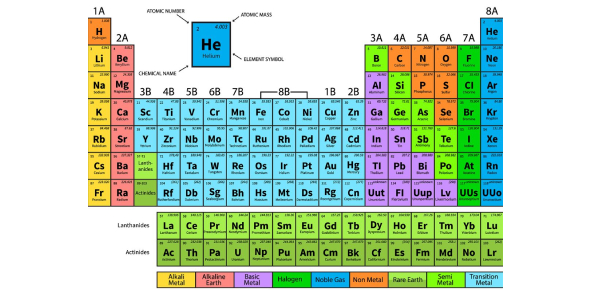# Periodic Table Quiz: Trivia Exam!

14 Questions | Total Attempts: 150Settings.

• 1.
Most of an atom is empty space.
• A.

True

• B.

False

• 2.
The energy of an electron depends on which energy level it is in.
• A.

True

• B.

False

• 3.
In an atom, an electron that is close to the nucleus is on the highest energy level.
• A.

True

• B.

False

• 4.
Elements are placed in the same column of the periodic table because they have the same number of outer electrons.
• A.

True

• B.

False

• 5.
The particles that make up an atom are ____.
• A.

Protons, nuclei, electrons

• B.

Elements, protons, neutrons

• C.

Protons, neutrons, nuclei

• D.

Protons, neutrons, electrons

• 6.
In an atom, electrons ____.
• A.

Are located in the nucleus

• B.

Are paired with neutrons

• C.

Circle outside the nucleus

• D.

Are always in the same place

• 7.
Dot diagrams are used to represent ____.
• A.

Protons

• B.

Outer electrons

• C.

Total electrons

• D.

Neutrons

• 8.
The energy levels of an atom are occupied by ____.
• A.

Electrons

• B.

Protons

• C.

Neutrons

• D.

Ions

• 9.
The maximum number of electrons in the second energy level of an atom is ____.
• A.

Two

• B.

Four

• C.

Eight

• D.

Ten

• 10.
Which element has a stable number of electrons in its outer energy level?
• A.

Neon

• B.

Hydrogen

• C.

Chlorine

• D.

Sulfur

• 11.
How many dots are shown in the electron dot diagram for calcium?
• A.

One

• B.

Two

• C.

Eight

• D.

Twenty

• 12.
Which of the following statements about the atom is false?
• A.

Atoms have a tiny nucleus in the center.

• B.

Protons and neutrons are located in the nucleus.

• C.

Electrons circle the nucleus like planets.

• D.

All atomic nuclei are positively charged.

• 13.
Which element is in the same family as magnesium?
• A.

Sodium

• B.

Sulfur

• C.

Potassium

• D.

Calcium

• 14.
Which statement is true of an element that has 5 protons and 5 electrons?
• A.

It has 5 neutrons.

• B.

It has an atomic mass of 10.

• C.

It has an atomic mass of 5.

• D.

It has an atomic number of 5.

Related TopicsBack to top